#Function Repository Resource:

# VizingEdgeColoring

Give a simple undirected graph a proper edge coloring using at most one color more than necessary

Contributed by: Daniel McDonald
 ResourceFunction["VizingEdgeColoring"][gra] finds a proper edge coloring of the simple undirected graph gra using at most one more color than necessary.

## Details and Options

Vizing’s theorem states that if G is a simple undirected graph having maximum degree Δ, then G has a proper (Δ+1) edge coloring. Here, a proper k edge coloring is a coloring of the edges of G from a palette of k colors such that no vertex is incident to two or more identically colored edges. Note that at least Δ colors are needed for a proper edge coloring of G, to provide enough distinct colors for edges incident to vertices of maximum degree. Combined with Vizing’s theorem, it follows that the edge chromatic number of G, which equals the minimum k such that G has a proper k edge coloring, is either Δ or Δ+1.
The proper edge coloring is returned in the form of an Association whose key-value pairs are edges with “color” an integer that is either Δ or Δ+1.

## Examples

### Basic Examples

Define a graph and find a proper edge coloring:

 In:=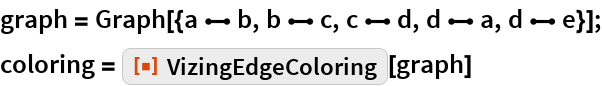Out=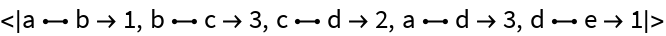Define a function for plotting a proper edge coloring:

 In:=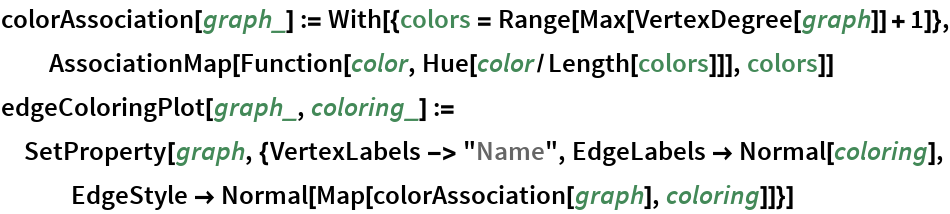Plot the proper edge coloring:

 In:=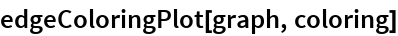Out=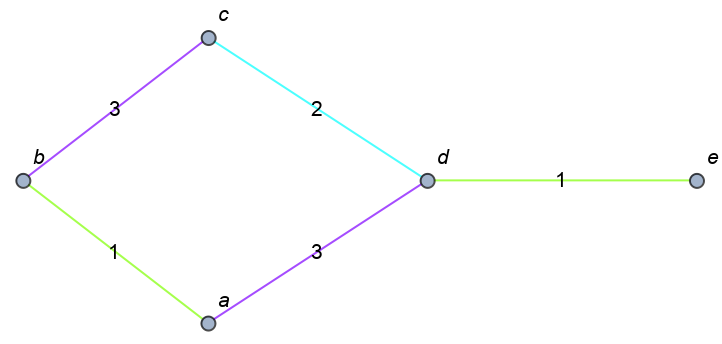Find a proper edge coloring for the Petersen graph:

 In:=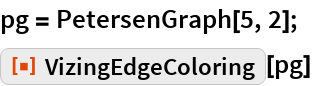Out=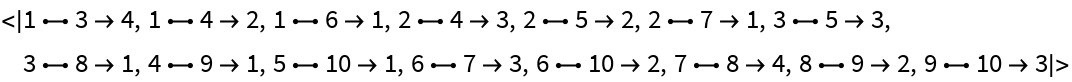Plot it:

 In:=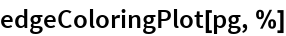Out=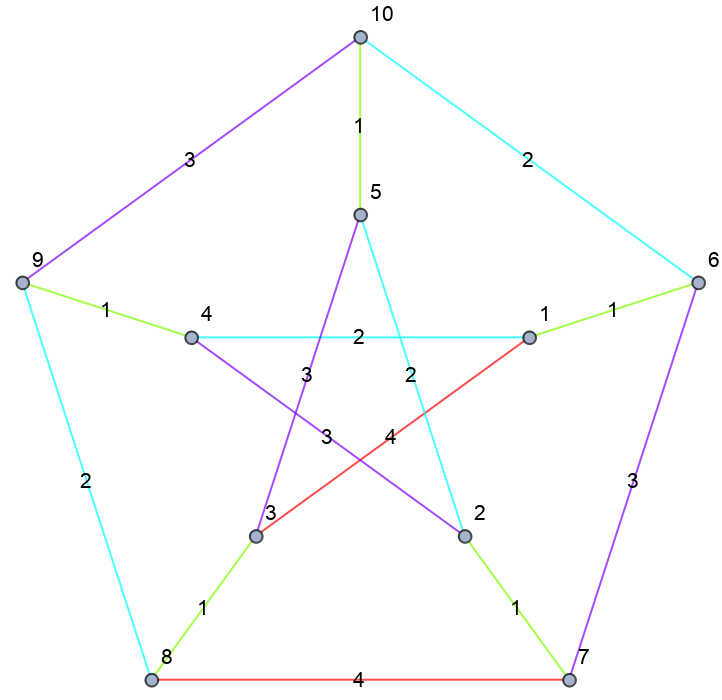## Requirements

Wolfram Language 11.3 (March 2018) or above